SSC  >  SSC CPO Mock Test (Quant Apt) 8

# SSC CPO Mock Test (Quant Apt) 8

Test Description

## 50 Questions MCQ Test SSC CPO & Constable - Mock Tests & Previous Year Papers | SSC CPO Mock Test (Quant Apt) 8

SSC CPO Mock Test (Quant Apt) 8 for SSC 2023 is part of SSC CPO & Constable - Mock Tests & Previous Year Papers preparation. The SSC CPO Mock Test (Quant Apt) 8 questions and answers have been prepared according to the SSC exam syllabus.The SSC CPO Mock Test (Quant Apt) 8 MCQs are made for SSC 2023 Exam. Find important definitions, questions, notes, meanings, examples, exercises, MCQs and online tests for SSC CPO Mock Test (Quant Apt) 8 below.
Solutions of SSC CPO Mock Test (Quant Apt) 8 questions in English are available as part of our SSC CPO & Constable - Mock Tests & Previous Year Papers for SSC & SSC CPO Mock Test (Quant Apt) 8 solutions in Hindi for SSC CPO & Constable - Mock Tests & Previous Year Papers course. Download more important topics, notes, lectures and mock test series for SSC Exam by signing up for free. Attempt SSC CPO Mock Test (Quant Apt) 8 | 50 questions in 30 minutes | Mock test for SSC preparation | Free important questions MCQ to study SSC CPO & Constable - Mock Tests & Previous Year Papers for SSC Exam | Download free PDF with solutions
 1 Crore+ students have signed up on EduRev. Have you?
SSC CPO Mock Test (Quant Apt) 8 - Question 1

### A man is 24 years older than his son. In two years, his age will be twice the age of his son. The present age of the son is :

Detailed Solution for SSC CPO Mock Test (Quant Apt) 8 - Question 1 Let the son's present age be x years
Then, man's present age = (x + 24) years
∴ (x + 24) + 2 = 2(x + 2)
⇒ x + 26 = 2x + 4
⇒ x = 22
SSC CPO Mock Test (Quant Apt) 8 - Question 2

### The roots of 2x²-6x+3=0 are

SSC CPO Mock Test (Quant Apt) 8 - Question 3

### If 1/x=1/y+1/z, then z equals

SSC CPO Mock Test (Quant Apt) 8 - Question 4

Three math classes : X, Y and Z take an algebra test
1. The average score of class X is 83
2. The average score of class Y is 76.
3. The average score of class Z is 85.
4. Average score of class X and Y is 79.
5. Average score of class Y and Z is 81.
What is the average score of classes X, Y, Z?

Detailed Solution for SSC CPO Mock Test (Quant Apt) 8 - Question 4

Let the number of students in class x, y & z be a, b and c respectively
Then,
Total of x = 83 a
Total of y = 76 b
Total of z = 85 c
And,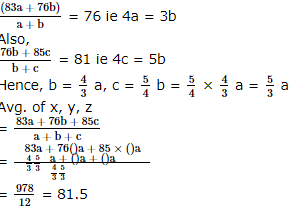SSC CPO Mock Test (Quant Apt) 8 - Question 5
For Rs 300 you can buy either packet-1 containing 9 paper weight and 7 ball pens or pacjet-II containing 6 paper weight and 13 ball pens. The price of each paper weight is
Detailed Solution for SSC CPO Mock Test (Quant Apt) 8 - Question 5 Let Price of each paper weight be Rs. x and ball Pens be Rs. y
9x + 7y = 300 .....(i)
6x + 13y = 300 .....(ii)
Solving (i) & (ii) we get
y = 12 & x = 24
SSC CPO Mock Test (Quant Apt) 8 - Question 6
A classroom has equal number of boys and girls.8 girls left to play Kho-Kho,leaving twice as many boys as girls in the classroom. What was the total number of girls and boys present initially?
Detailed Solution for SSC CPO Mock Test (Quant Apt) 8 - Question 6 Let number of boy and girls in class are x & y
Then, x = 2 (y - 8)
or x = 2y - 16
again, x = y
or 2y - 16 = y
or y = 16
Total Number of boys & girls are 32
SSC CPO Mock Test (Quant Apt) 8 - Question 7

Area of a square field is 1 hectare. The length of another square field is 1% more than the length of this square field. The difference in their areas is

Detailed Solution for SSC CPO Mock Test (Quant Apt) 8 - Question 7

1 hectare = 10000 m2
Area of a square field = 10000 m2
let length of a square field be x m
then x2 = 10000
x = 100m
length of other square field = 100 + 100 x 1/100 = 101
Area of Another square field = (101)2 = 10201 m2
∴ Difference in their areas = 10201 - 10000
= 201 m2

SSC CPO Mock Test (Quant Apt) 8 - Question 8

A circular running path is 726 metres in circumference. Two men start from the same point and walk in opposite directions at the speed of 3.75 km. per hour and 4.5 km per hour respectively. Then when will they meet for first chance:

Detailed Solution for SSC CPO Mock Test (Quant Apt) 8 - Question 8

The two men walk in opposite directions @ 62.5 metres/minute and 75 metres/minute respectively
∴ They will meet for the first time after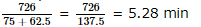SSC CPO Mock Test (Quant Apt) 8 - Question 9
In Arun's opinion, his weight is greater than 65 kg but less than 72 kg. His brother does not agree with Arun and he thinks that Arun's weight is greater than 60 kg but less than 70 kg. His mother's view is that his weight cannot be greater than 68 kg. If all of them are correct in their estimation, what is the average of different probable weights of Arun?
SSC CPO Mock Test (Quant Apt) 8 - Question 10
What is the perimeter of the rectangle ABCD when AD = 3, and BD = 5
SSC CPO Mock Test (Quant Apt) 8 - Question 11
The foot of th eperpendicular from the point (2, 4) upon x + y = 1 is
SSC CPO Mock Test (Quant Apt) 8 - Question 12
A sum of money invested at Compound Interest amounts to Rs 640 at the end of first year and Rs 676 at the end of second year. The sum of money is closest to
SSC CPO Mock Test (Quant Apt) 8 - Question 13
What is the smallest number that has to be added to 710 so that the sum is a perfect cube?
SSC CPO Mock Test (Quant Apt) 8 - Question 14
A shopkeeper marks his sarees at 20% above the cost price and allows the purchaser a discount of 10% for cash buying. What profit per cent does he make?
SSC CPO Mock Test (Quant Apt) 8 - Question 15
Which is the largest among the following fractions? 5/8, 2/3, 7/9, 3/5, 4/7
SSC CPO Mock Test (Quant Apt) 8 - Question 16

A polygon has 5 diagonals. The polygon is

Detailed Solution for SSC CPO Mock Test (Quant Apt) 8 - Question 16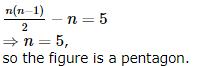SSC CPO Mock Test (Quant Apt) 8 - Question 17

AB and CD are two straight lines. Transversal EF intersects them as shown in the given figure. If b = c then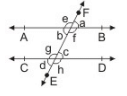Detailed Solution for SSC CPO Mock Test (Quant Apt) 8 - Question 17

Clearly x + y = 180°

SSC CPO Mock Test (Quant Apt) 8 - Question 18
PT is a tangent and PAB is a secant to the circle. If PT = 5 cm and PA = 4 cm then the length of AB is
SSC CPO Mock Test (Quant Apt) 8 - Question 19
The sides of a triangle ABC are 21 metres, 20 metres and 13 metres respectively. The longest side is AB. If a perpendicular CD is drawn from C on AB, then the difference of the area (in sq. metres) of the 2 triangles ACD and BCD will be
SSC CPO Mock Test (Quant Apt) 8 - Question 20
If two numbers are in the ratio of 2:3 and the product of their HCF and LCM is 33750,then the sum of the numbers is
SSC CPO Mock Test (Quant Apt) 8 - Question 21

Two villages are 2 km apart.If the angles of depression of these villages when observed from a plane are found to be 45°and 60° respectively,then the height of the plane is

SSC CPO Mock Test (Quant Apt) 8 - Question 22
An integer is 10 more than its one-third. The integer is
SSC CPO Mock Test (Quant Apt) 8 - Question 23

If x% of y is equal to z, what percent of z is x?

SSC CPO Mock Test (Quant Apt) 8 - Question 24

A boy,after giving away 80% of his pocket money to one companion and 6% of the remainder to another, has 47 paise left with him. How much pocket money did the boy have in the begining ?

Detailed Solution for SSC CPO Mock Test (Quant Apt) 8 - Question 24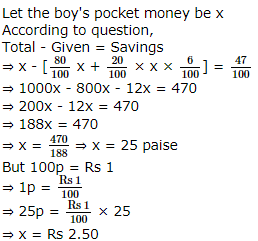SSC CPO Mock Test (Quant Apt) 8 - Question 25
Taps A and B can fill a bucket in 12 minutes and 15 minutes respectively. If both are opened and A is closed after 3 minutes, how much further time would it take for B to fill the bucket?
SSC CPO Mock Test (Quant Apt) 8 - Question 26
What per cent of selling price would be 34% of cost price if gross profit is 26% of the selling price?
SSC CPO Mock Test (Quant Apt) 8 - Question 27
A merchant bought 60 sheeps at Rs 120 per sheep. He sold 40 of them at Rs 150 each. 10 of them died. What should be the selling price of the remaining sheeps if he wants a profit of Rs 800?
SSC CPO Mock Test (Quant Apt) 8 - Question 28
If Rs. 1066 are divided among A, B, C and D such that A : B = 3 : 4, B : C = 5 : 6 and C : D = 7 : 5, who will get the maximum
SSC CPO Mock Test (Quant Apt) 8 - Question 29

In a ΔABC, A=90º . Then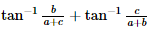is equal to

SSC CPO Mock Test (Quant Apt) 8 - Question 30
A and B have monthly incomes in the ratio 5:6 and monthly expenditures in the ratio 3:4. If they save Rs 1800 and Rs 1600 respectively,find the monthly income of B.
SSC CPO Mock Test (Quant Apt) 8 - Question 31
In a G.P. if the (m + n)th term is p and the (m - n) th term is q, then its mth term is
SSC CPO Mock Test (Quant Apt) 8 - Question 32
Rs 5000 earned Rs 300 interest in 2 yrs. The rate of interest is
SSC CPO Mock Test (Quant Apt) 8 - Question 33

(169-144)¹∕² ÷ (64+36)¹∕²=?

SSC CPO Mock Test (Quant Apt) 8 - Question 34
A man reduces his speed a two-third to walk a distance and consequently becomes late by one hour. With his usual speed, he covers the same distance in
SSC CPO Mock Test (Quant Apt) 8 - Question 35
P can complete a work in 12 days working 8 hours a day. Q can complete the same work in 8 days working 10 hours a day. If both P and Q work together, working 8 hours a day, in how many days can they complete the work?
SSC CPO Mock Test (Quant Apt) 8 - Question 36

The angle subtended at the centre of a circle of radius 3 m by the arc measuring 1 m is:

SSC CPO Mock Test (Quant Apt) 8 - Question 37

The value of cos²θ+sec²θ is always

SSC CPO Mock Test (Quant Apt) 8 - Question 38
If cos (A - B)=3/5 tan A tan B=2, then
SSC CPO Mock Test (Quant Apt) 8 - Question 39

Into a circular drum of radius 4.2 m and height 3.5 m how many full bags of wheat can be emptied if space required for each wheat bags is 2.1m3.

Detailed Solution for SSC CPO Mock Test (Quant Apt) 8 - Question 39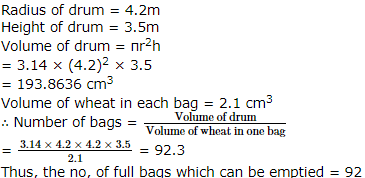SSC CPO Mock Test (Quant Apt) 8 - Question 40

A hall is 15 m long and 12 m broad. If the sum of the areas of the floor and the ceiling is equal to the sum of areas of the four walls, the volume of the hall is :

Detailed Solution for SSC CPO Mock Test (Quant Apt) 8 - Question 40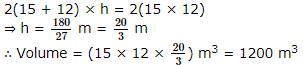SSC CPO Mock Test (Quant Apt) 8 - Question 41

A cylinder with base radius of 8 cm and height of 2 cm is melted to form a cone of height 6 cm. The radius of the cone will be :

Detailed Solution for SSC CPO Mock Test (Quant Apt) 8 - Question 41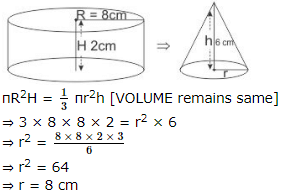SSC CPO Mock Test (Quant Apt) 8 - Question 42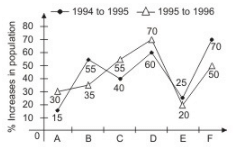Above graph shows the percentage growth in population in six states A, B, C, D, E, and F from 1994 to 1995 and 1995 to 1996. Study the graph and answer their questions.

Q. Population of State 'F' in 1995 was approximately what percent of its population in 1996 ?

Detailed Solution for SSC CPO Mock Test (Quant Apt) 8 - Question 42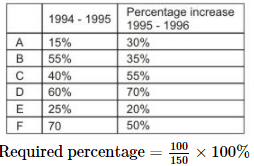= 66.66%
≈67%
[Let population in 1995 be 100 then population in 1996 is 150]

SSC CPO Mock Test (Quant Apt) 8 - Question 43Above graph shows the percentage growth in population in six states A, B, C, D, E, and F from 1994 to 1995 and 1995 to 1996. Study the graph and answer their questions.

Q. If the population of State 'B' in the year 1994 was 5 lakh, what was approximately its population in the year 1996?

Detailed Solution for SSC CPO Mock Test (Quant Apt) 8 - Question 43

Population of B in 1996 = 5 × 1.55 × 1.35 lakh = 10.4625 lakhs
≈10.5 lakhs

SSC CPO Mock Test (Quant Apt) 8 - Question 44Above graph shows the percentage growth in population in six states A, B, C, D, E, and F from 1994 to 1995 and 1995 to 1996. Study the graph and answer their questions.

Q. If the population of States C and D in 1995 are in the ratio 2 : 3 respectively and the population of State 'C' in 1994 was 2.5 lakhs, what was the population of State D in 1995 ?

Detailed Solution for SSC CPO Mock Test (Quant Apt) 8 - Question 44

Popualtion of C : Population of D = 2 : 3

Hence Population of D in 1994
= 3.75 × = 6 lakh
Population of D in 1995 = 3.75 lakhs

SSC CPO Mock Test (Quant Apt) 8 - Question 45Above graph shows the percentage growth in population in six states A, B, C, D, E, and F from 1994 to 1995 and 1995 to 1996. Study the graph and answer their questions.

Q. In 1994 the population of States B and D are equal and the population of State B in 1996 is 20.925 lakhs. What approx. was the population of State 'D' in 1996 ?

Detailed Solution for SSC CPO Mock Test (Quant Apt) 8 - Question 45

Let population of B in 1994 be x
Then x × 1.55 × 1.35 = 20.925 lakhs
⇒ x = 10 lakhs
As in 1994, population of D = Population of B Hence Population of D in 1994 is 10 lakhs Population of D in 1996 is = 10 × 1.6 × 1.7 lakh = 27.2 lakh
≈27 lakhs

SSC CPO Mock Test (Quant Apt) 8 - Question 46

The pie chart given below shows the funding arrangements for National Highways Development Projects-Phase 1. Study the chart carefully to answer these questions.
Funding arrangements for NHDP-I(total cost: Rs.30,000 Crores)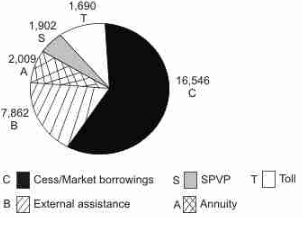Q. Near about 25% of the funding arrangement is through

Detailed Solution for SSC CPO Mock Test (Quant Apt) 8 - Question 46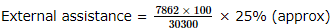SSC CPO Mock Test (Quant Apt) 8 - Question 47

The pie chart given below shows the funding arrangements for National Highways Development Projects-Phase 1. Study the chart carefully to answer these questions.
Funding arrangements for NHDP-I(total cost: Rs.30,000 Crores)Q. The angle of the segment formed at the center of the pie chart, representing Cess/Market borrowings is approximately

Detailed Solution for SSC CPO Mock Test (Quant Apt) 8 - Question 47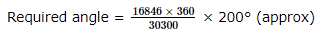SSC CPO Mock Test (Quant Apt) 8 - Question 48

The pie chart given below shows the funding arrangements for National Highways Development Projects-Phase 1. Study the chart carefully to answer these questions.
Funding arrangements for NHDP-I(total cost: Rs.30,000 Crores)Q. If the toll is to be collected through an outsourced agency by allowing a maximum of 10% commission. How much amount should be permitted to be collected by the outsourced agency, so that the project is supported with Rs.1,690 Crores?

Detailed Solution for SSC CPO Mock Test (Quant Apt) 8 - Question 48

Amount required to be permitted = 1690/0.9 = Rs 1878 crore

SSC CPO Mock Test (Quant Apt) 8 - Question 49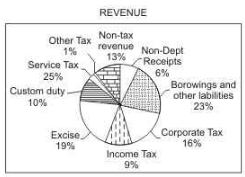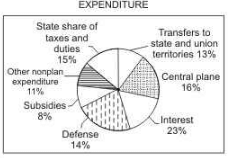The pie charts show estimated revenue and expenditure budgets for 2004-2005 for country X.
Notes: 1. Corporate tax revenues increased by Rs.2545 crore in the budget estimates for 2004-2005 over the estimates for 2003-2004.
2. Service tax brings in Rs.3990crore according to the budget for 2004-2005.
3. Total expenditure on defence increased by a whopping 28 percent from Rs.6030crore in 2003-2004 to Rs.7700crore in 2004-2005.

Q. The revenue generated by Custom duty, Income tax and Corporate tax according to the budget for 2004-2005 is.

SSC CPO Mock Test (Quant Apt) 8 - Question 50The pie charts show estimated revenue and expenditure budgets for 2004-2005 for country X.
Notes: 1. Corporate tax revenues increased by Rs.2545 crore in the budget estimates for 2004-2005 over the estimates for 2003-2004.
2. Service tax brings in Rs.3990crore according to the budget for 2004-2005.
3. Total expenditure on defence increased by a whopping 28 percent from Rs.6030crore in 2003-2004 to Rs.7700crore in 2004-2005.

Q. What is the percentage increase in the revenue generated by corporate tax is 2004-2005 over 2003-2004 according to the budgets?

## SSC CPO & Constable - Mock Tests & Previous Year Papers

1 docs|63 tests
 Use Code STAYHOME200 and get INR 200 additional OFF Use Coupon Code
Information about SSC CPO Mock Test (Quant Apt) 8 Page
In this test you can find the Exam questions for SSC CPO Mock Test (Quant Apt) 8 solved & explained in the simplest way possible. Besides giving Questions and answers for SSC CPO Mock Test (Quant Apt) 8, EduRev gives you an ample number of Online tests for practice

1 docs|63 tests